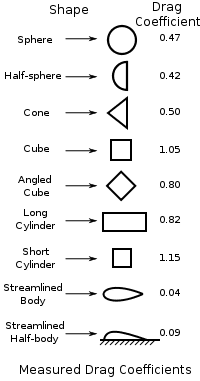# Force of Drag

Hi

I know what pressure and friction drag are, I am having a hard time trying to find out how can you calculate the coefficient of drag for them?
I think i have came across an equation for it: F$_{D}$ = .5*ρ*v$^{2}$*A*C$_{D}$
where F$_{D}$ is the force of drag, ρ is density, v is velocity, A is the contact area and C$_{D}$ is the coefficent of drag

is there any other equation used to calculate C$_{D}$?

Cd is the ratio of the drag of the body in question when compared with a brick of the same frontal dimensions. It is a function of the geometry of the object - more streamlined, lower Cd.

A brick* has a Cd of 1 (by definition). A modern car is typically a Cd of ~0.3. A car with a Cd of 0.25 would be one of the more streamlined car bodies (at this current time). An aerofoil shape is ~0.1, or much less, all dependent on the geometry of the object. You can simulate for it, but ultimately you measure it in a wind tunnel.

*(That is to say, the assumption is that you are simply accelerating all the air infront of the moving object up to the same speed as the object passing through it.)Cd is the ratio of the drag of the body in question when compared with a brick of the same frontal dimensions.

So for example if you had a double decker bus, you would put that (or a scaled down version of it) in a wind tunnel and get the drag of the body and then get a brick of the same size in the the wind tunnel and get the drag of the body and then get the ratio of them?

how would it be done for a plane or a car when the frontal dimensions are not easily found?

I would be guessing, but these days if they wanted the cross-sectional area I presume they'd get a CAD model of the frontal view of the thing, and get the computer to work out the area. If you wanted to do this for yourself you could just take a photo, with a meter rule in the picture to act as a graticule, and count up the squares!

There is no need to measure the Cd of a brick! If you look at your equation, it is simply a calculation based on the kinetic energy of a given amount of displaced air, accelerated to the velocity. To determine the drag, I expect they'd just measure the force on the object at a given wind speed and back-calculate the equivalent 'power' that would be required (force x velocity), which then provides an equivalence to how much air would need to be accelerated (that is, if the air were stationary and pushed out of the way by the moving object).

I expect there are non-linearities of Cd according to the airspeed and the particular nature of the design, but I'm sure if an engineer wants the Cd accurately for some reason then they'd derive it for a given velocity through the air.